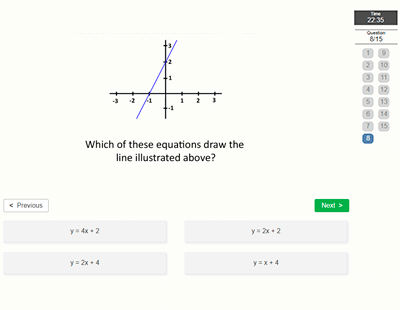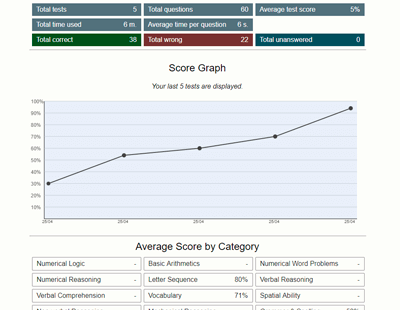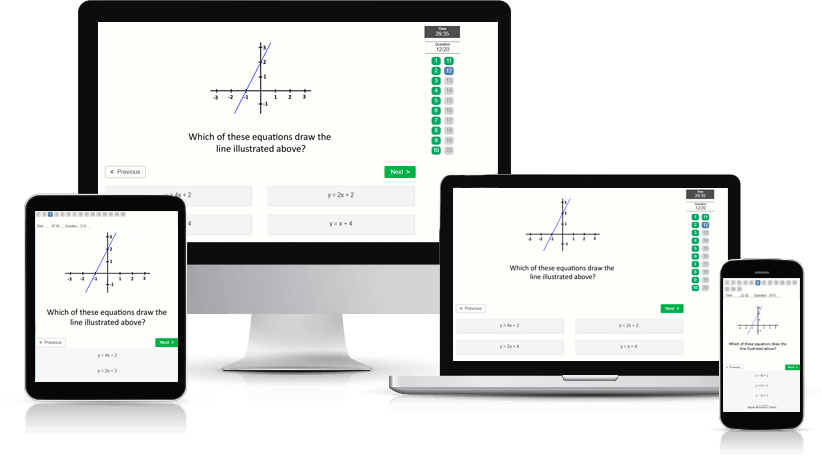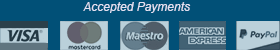﻿ Mathematical Knowledge Test - Aptitude-Test.com

# Mathematical Knowledge Test

In mathematical knowledge your knowledge of mathematics on a high school level will be tested. Calculators are normally allowed during these tests, and using pen and paper is highly recommended.

What questions can I expect?
In a math knowledge test you are required to solve mathematical problems and answer questions regarding mathematical theory. When facing a mathematical knowledge test, we suggest that you brush up on the following topics:

• Order of Operation
• Types of numbers
• Decimals
• Percentages
• Interest
• Factorial
• Exponents
• Pythagoras
• 2D and 3D Geometric figures
• Names of common figures
• Volume and area
• Circumference
• Linear equations

Where are Mathematical Knowledge tests used?
These are most commonly used as part of school admission exams. The mathematical knowledge test is also part of the ASVAB test.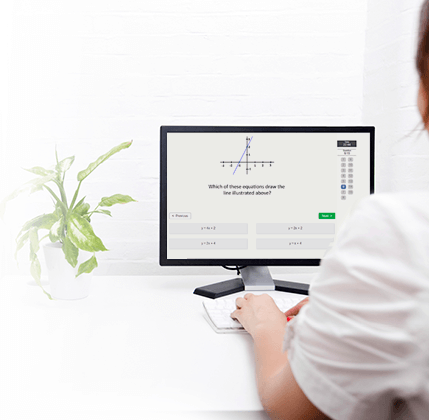## Practice Mathematical Knowledge Test

Try a free Mathematical Knowledge Test.
This free practice test contains 6 test questions and has a time limit of 6 minutes.

##### Would you like to improve your test score? Practice smart with a Test Prep Account.

Practice on 58 Mathematical Knowledgeand a total of 426 numerical aptitude test questions with detailed description and score statistics.

The many features of a Test Prep Account:

• Preparation software developed under a didactic and methodical perspective
• High-quality practice test questions
• Clearly explained solutions
• Accessible on all devices
• Detailed score data and progression charts
• Reference scores to compare your performance against others
• Training Assistant
• Practice mode (feedback after every question and no time limit)